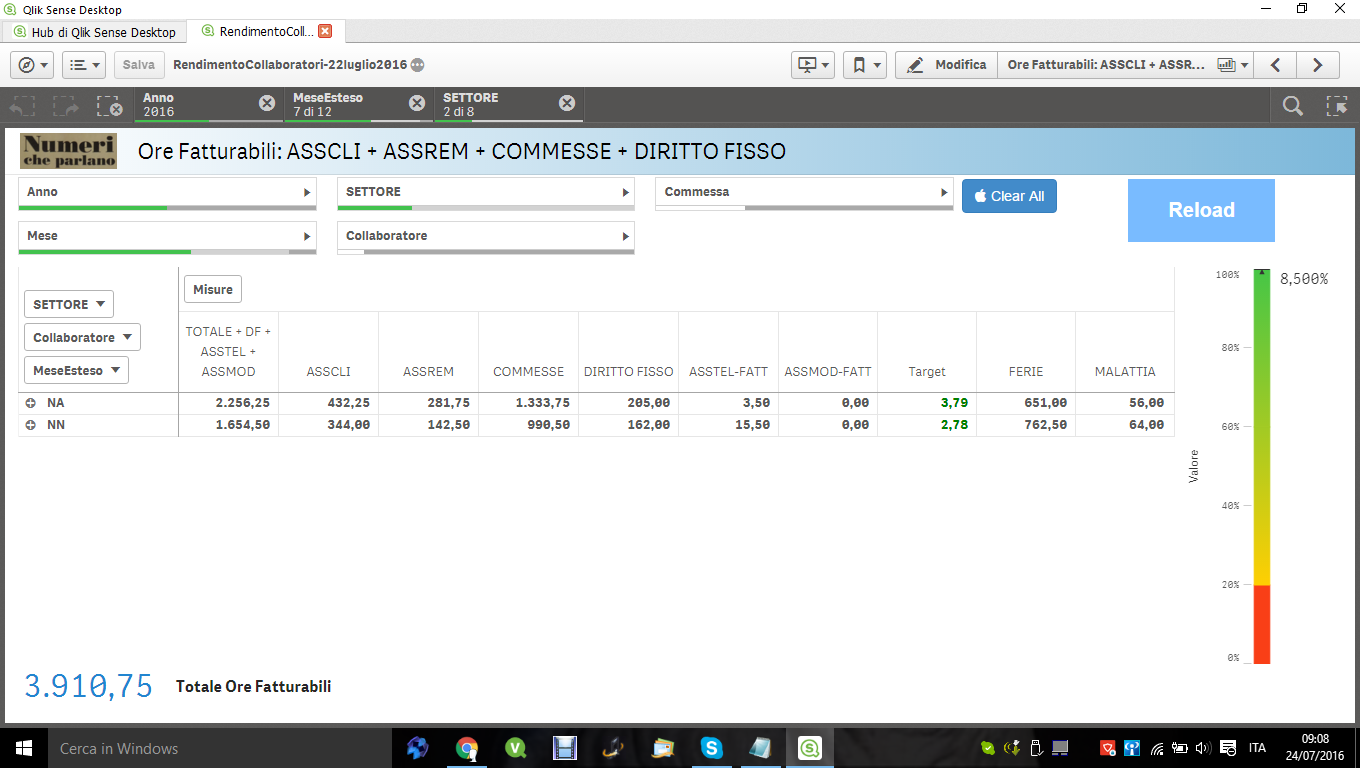# New to Qlik Sense

Discussion board where members can get started with Qlik Sense.

Announcements
Coming your way, the Qlik Data Revolution Virtual Summit. October 27-29. REGISTER
cancel
Showing results for
Did you mean:
HighlightedPartner

## target

Hi Community,

how to prevent calculation of the formula is applied to the total row (red box)

(Sum(DIRFIS)

+ sum({<Commessa = {"=KeepChar(Commessa, '0123456789') * 1 > 10"}>} [TotOreLavorate])

+ sum({<Commessa = {'ASSCLI', 'ASSREM'}>} [TotOreLavorate])

+ sum({<CTRAAN = {'N',""},Commessa = {'ASSTEL'}>} [TotOreLavorate])

+ sum({<CTRAAN = {'T',""},Commessa = {'ASSMOD'}>} [TotOreLavorate]))

/ (85 * (count(MeseEsteso)))9 Replies
HighlightedMVP

May be use of Dimensionality() or SecondaryDimensionality() to get this done.

How to use - Dimensionality()

The second dimension... or how to use secondarydimensionality()

HighlightedPartner

Hi

sin, use Qlik sense

HighlightedMVP
HighlightedPartner

Hi,

Unfortunately I have not figured out how to activate the function

HighlightedMVP

Would you be able to share a sample to help you better?

HighlightedPartner

Hi Sunny,

the best I can give you is the image attachedNN - NA lines are total lines and should have the value 0

I know it's my interest give my app but it includes sensitive data and sql connections . To protect the privacy I can not disclose certain information

HighlightedSpecialist

Have you considered using a straight table? With a straight table you can have the field NA/NN but it won't group by it and you can avoid the total row.

HighlightedPartner

HI,

I understand the suggestion , but I would like to take the path indicated by Sunny . I hope I can give an example to follow

HighlightedPartner

It was very simple . I found an example and I managed to figure out how to make it happen .
The dimantionality function allows you to have the level for each line and then just an IF to determine if the level should be tested or not.

if the level is 1, then I put " - " if the level is 2 I apply the formula .

if (Dimensionality() = 2,(Sum(DIRFIS)

+ sum({<Commessa = {"=KeepChar(Commessa, '0123456789') * 1 > 10"}>} [TotOreLavorate])

+ sum({<Commessa = {'ASSCLI', 'ASSREM'}>} [TotOreLavorate])

+ sum({<CTRAAN = {'N',""},Commessa = {'ASSTEL'}>} [TotOreLavorate])

+ sum({<CTRAAN = {'T',""},Commessa = {'ASSMOD'}>} [TotOreLavorate]))

/ (85 * (count(MeseEsteso))), '-')